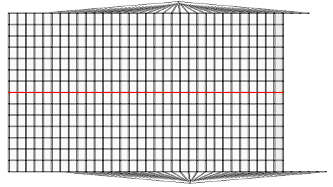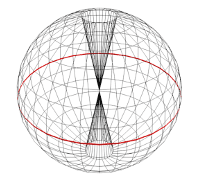The Principles of the Trinary Universe Chapter 1.03:
Light is not a Straight Line
by
Jeffrey Scott Flesher Medically Retired United States Air Force Staff Sergeant Last Update: 14 January 2019

### Chapter 1.03:Light is not a Straight Line

When I started counting Numbers to keep my mind busy, I did not just pick any numbers, I had to figure out vectors for Light to travel in straight lines, always looking for the most optimized way of calculating it, which over my lifetime lead me to learn every recorded form of Math used to calculate vectors in a straight line, but math like y = mx + b is not optimized for large distances where x is measured in Billions of Light Years, and y and b is unknown, since I was trying to figure out if there is a beginning and end point to that line, which eventually lead me to use Multidimensional Math, which I visualized as geometric shapes: a rectangle represented the path of the straight line, it boxes it in, triangles or pyramids represented the single photon of Light, when the Light was Solid or Brightest, it was inside of a Sphere, as it changed states to a Darker intensity, the Sphere would twist like a figure 8, so Sphere was like a Toroid, and I envisioned as being a Sphere with a hole through it, from pole to pole, so Trinary Engines  and Atom's have this Shape, not sure if they actually exist since I have never seen one, but I know they exist because the Atom must have a way to disappear, it is not Magic, so like a Wizard, I must describe what the Atom would have to look like if it could disappear with an explanation, so it looked like two triangles pointing at each other, or one Twisted Spherical Toroid which I visualize as two triangles joined at the tips, so it is a figure 8, its just how we model constructs in 2D and 3D, then it would turn inside out and appear as a Sphere with nothing in it, this math was clearly a visualization of how the Light changes states in terms of images and it used only 3 shapes: Rectangle, Triangle and Circle, so it was the most optimized way of visualizing vectors in a straight line, since each object represented a state change, and it was bond to the Rectangle, it also had relationships with each other, but needless to say, a straight line is a boring path to take, but I realized that in Real 3 Dimensional Space, this path would not travel through the Universe on a straight line, as if Space was like a sheet of paper that represented the Universe, and a straight line drawn down the middle of its width wise, as shown in: Illustration 1.01: Spherical Light , with a line representing the Light path as flat, knowing it is not flat, since it actually goes around the entire Sphere, my equation was setup to figure out if the paper could be seamed together into a sphere, such that the straight line would form a circle that ran around the sphere as shown in: Illustration 1.02: Circular Spherical Light, shown as a line representing the Light path in a Circle.Illustration 1.01: Flat Spherical Light Full SizeIllustration 1.02: Circular Spherical Light Return 1 Full Size

I wanted to know if this was possible for Light to form a magnetic line of flux and curve back to form a complete path, so this Circle is an unknown or undefined shape, it is a loop, elliptical or oval shaped like a magnet, same principle as a magnetic field, because I figured out that expanding infinitely on a straight line was not probable, because it would not have a beginning or an end point, although this went along with the science of my time, I did not believe that space was like water in an infinite universe and could flow infinitely in one direction, and the more I thought about it the more convinced I became that it was not what was going on, in fact I know for a fact that this is not what we see when we look into deep space, in fact I know if we look far enough, we would see ourselves from behind, not that we would ever have telescopes that powerful, and it would take over 13 billion years for the Light to travel that far, so it was the time it would take to prove such a concept quickly became unmanageable, but I saw the universe compartmentalized into corridors of space, which lead me to think that there are actually multiple corridors of Space in the Universe, each was in the shape of a sphere and although you could see other corridors you could not get from one corridor into another, because what we perceive as a straight line, is actually just a circle that has a very large radius of let us say 13 to 16 Billion Light years, which is a number I thought about for a long time, so it is not random or made up, but it could be between 13 and 16 Billion Light years, but it would be a miracle if I was right about any number I chose, so the number is just an educated guess, it all depends on the Frequency of Light, that is why it has such a wide gap of some 3 Billion Light Years, but Newton was the one always wondering how far we could see with better telescopes, and we still use the one he invented, and it has not changed much since he looked through it over 3 Centuries ago, sure we have bigger telescopes with better optics, and all the electronics we have to go with it, but the method of collecting Light with a Mirror and Lens is not new, and the physics of it never changes even though the technology does.